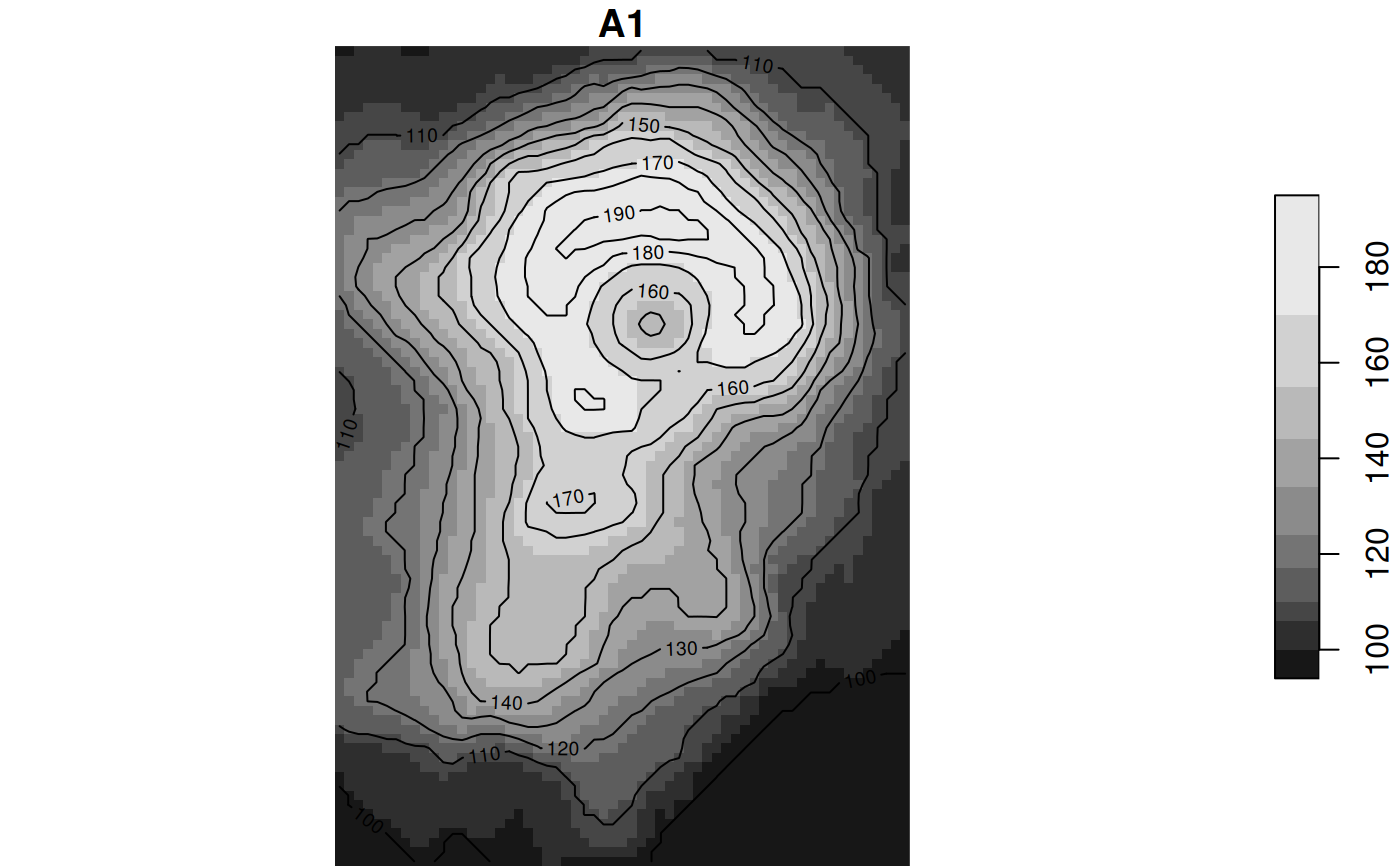plot contours of a stars object

# S3 method for stars
contour(x, ...)

## Arguments

x object of class stars other parameters passed on to contour

## Details

this uses the R internal contour algorithm, which (by default) plots contours; st_contour uses the GDAL contour algorithm that returns contours as simple features.

## Examples

d = st_dimensions(x = 1:ncol(volcano), y = 1:nrow(volcano))
r = st_as_stars(t(volcano))
r = st_set_dimensions(r, 1, offset = 0, delta = 1)
r = st_set_dimensions(r, 2, offset = 0, delta = -1)
plot(r, reset = FALSE)